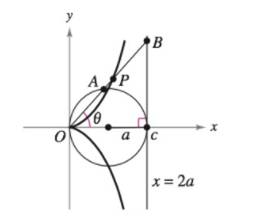Chapter 10, Problem 15PS

Chapter
Section
Textbook Problem

# Cissoid of Diocles Consider a circle of radius a tangent to the y-axis and the line x = 2 a , as shown in the figure. Let A be the point where the segment OB intersects the circle, where point B lies on the line x = 2 a . The cisoid of Diocles consists of all points P such that O P = A B .(a) Find a polar equation of the cissoid.(b) Find a set of parametric equations for the cissoid that does not contain trigonometric functions.(c) Find a rectangular equation of the cissoid.Figure for 15

(a)

To determine

To calculate: The polar equation of the cissoid.

Explanation

Given: The cissoids contains the points such that for the provided figure OP=AB.

Calculation:

Consider the triangle OCB.

cosθ=2aOBOB=2asecθ

Now consider triangle OAC

(b)

To determine

To calculate: The parametric equations of the cissoids without the trigonometric functions.

(c)

To determine

To calculate: The rectangular equation of the cissoid.

### Still sussing out bartleby?

Check out a sample textbook solution.

See a sample solution

#### The Solution to Your Study Problems

Bartleby provides explanations to thousands of textbook problems written by our experts, many with advanced degrees!

Get Started

#### In Exercises 9 and 10, find the limit. limx3(2x23x+4)

Calculus: An Applied Approach (MindTap Course List)

#### Write the sum in sigma notation. 12. 3+4+5+6+7

Single Variable Calculus: Early Transcendentals, Volume I

#### True or False: The x-intercepts are the values of x for which f(x) = 0.

Study Guide for Stewart's Single Variable Calculus: Early Transcendentals, 8th

#### The harmonic series is: 1 + 2 + 3 + 4 + …

Study Guide for Stewart's Multivariable Calculus, 8th